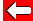# Quadratic Equations TestNow would be a good time for you to test what you have learned. Work out the solutions to each quadratic equation below:
 x² + 7x + 10 = 0. x² + x - 30 = 0. x² - 10x - 24 = 0.
Hints: Try to factorise each equation first. Be very careful with minus signs! If you get stuck, find a, b and c and plug them in the solution formula!

When you have written down your answers, check them on the next page...Go back a pageMaths MenuGo to next page